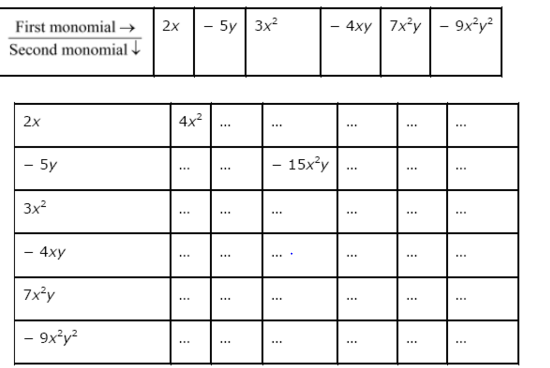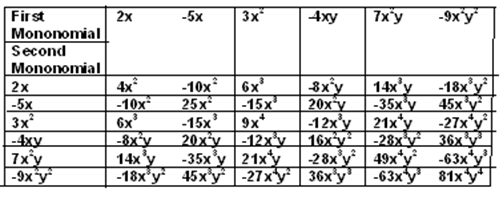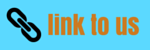# Ncert Solutions for Algebraic Expressions and Identities Class 8 Maths Chapter 9 Exercise 9.2

In this page we have NCERT Solutions for Algebraic Expressions and Identities Class 8 Maths Chapter 9 for Exercise 9.2 . Hope you like them and do not forget to like , social share and comment at the end of the page.
Question 1
Find the product of the following pairs of monomials.
(i) 4, 7p
(ii) – 4p, 7p
(iii) – 4p, 7pq
(iv)  4p3, – 3p
(v) 4p, 0
1. 4 x 7 p = 28p
2. - 4p x 7p = -28p2
3.  - 4p x 7pq = -28p2q
4. 4p3 x - 3p = -12p4
5. 4p x 0 = 0
Question 2
Find the areas of rectangles with the following pairs of monomials as their lengths and breadths respectively.
1. (p, q)
2. (10m, 5n)
3. (20x2 5y2)
4. (4x, 3x2)\
5. (3mn, 4np)
Answer: Now we know that
Area of rectangle = Length x breadth
So it is multiplication of monomials
(i) p x q = pq
(ii)10m x  5n = 50mn
(iii) 20x2 x  5y2 =  100x2y2
(iv) 4x x 3x2 = 12x3
(v)3mn x  4np = 12mn2p
Question 3.
Complete the following table of products:Question 4
Obtain the volume of rectangular boxes with the following length, breadth and height respectively.
1. 5a, 3a2, 7a8
2.  (2p, 4q, 8r)
3. xy, 2x2y, 2xy2
4. (a, 2b, 3c
Answer: We know that volume of rectangular box is given by Volume = length x  breadth x  height
(i) 5a x 3a2 x 7a8 = 105a11
(ii) 2p x 4q x 8r = 64pqr
(iii) xy x 2x2y x 2xy2 = 4x4y4
(iv) a x  2b x 3c = 6abc
Question 5
Obtain the product of
1. xy,  yz, zx
2. a, – a2 a3
3. 2, 4y, 8y2 16y3
4. a, 2b, 3c, 6abc
5. m, – mn, mnp
(i) x2y2z2
(ii) –a5
(iii) 1024y6
(iv) 36a2b2c2
(v) –m3n2p